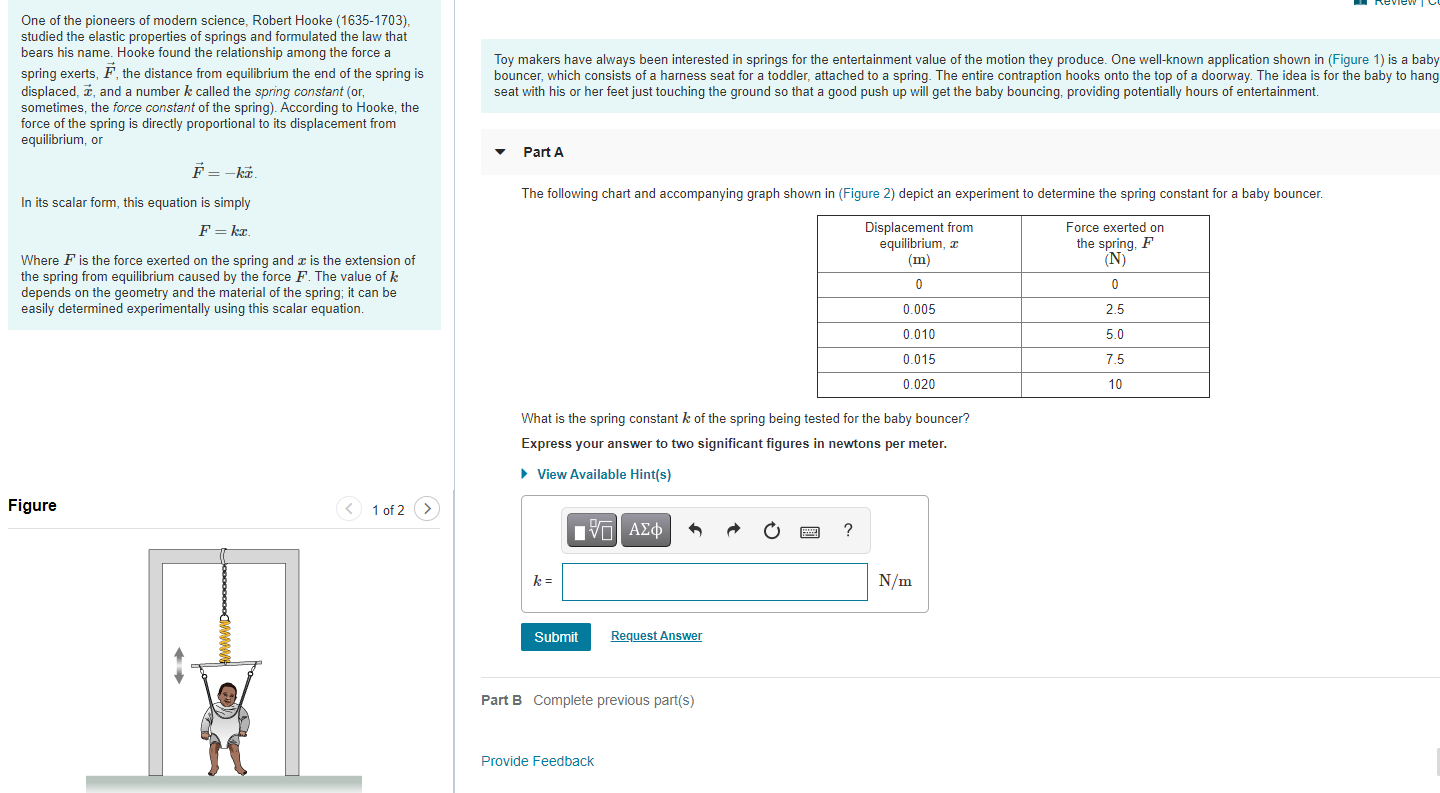# Question One of the pioneers of modern science, Robert Hooke (1635-1703), studied the elastic properties of springs and formulated the law that bears his name. Hooke found the relationship among the force a spring exerts, F, the distance from equilibrium the end of the spring is displaced, ž, and a number k called the spring constant (or, sometimes, the force constant of the spring). According to Hooke, the force of the spring is directly proportional to its displacement from equilibrium, or Toy makers have always been interested in springs for the entertainment value of the motion they produce. One well-known application shown in (Figure 1) is a baby bouncer, which consists of a harness seat for a toddler, attached to a spring. The entire contraption hooks onto the top of a doorway. The idea is for the baby to hang seat with his or her feet just touching the ground so that a good push up will get the baby bouncing, providing potentially hours of entertainment. Part A F=-ka. The following chart and accompanying graph shown in (Figure 2) depict an experiment to determine the spring constant for a baby bouncer. In its scalar form, this equation is simply F = kx Displacement from equilibrium, 3 (m) Force exerted on the spring, F (N) Where F is the force exerted on the spring and is the extension of the spring from equilibrium caused by the force F. The value of k depends on the geometry and the material of the spring, it can be easily determined experimentally using this scalar equation. 0 0 0.005 2.5 0.010 5.0 0.015 7.5 0.020 10 What is the spring constant k of the spring being tested for the baby bouncer? Express your answer to two significant figures in newtons per meter. View Available Hint(s) Figure 1 of 2 O AO ? k= N/m Submit Request Answer Part B Complete previous part(s) Provide Feedback